Ex 5.2

Chapter 5 Class 10 Arithmetic Progressions
Serial order wise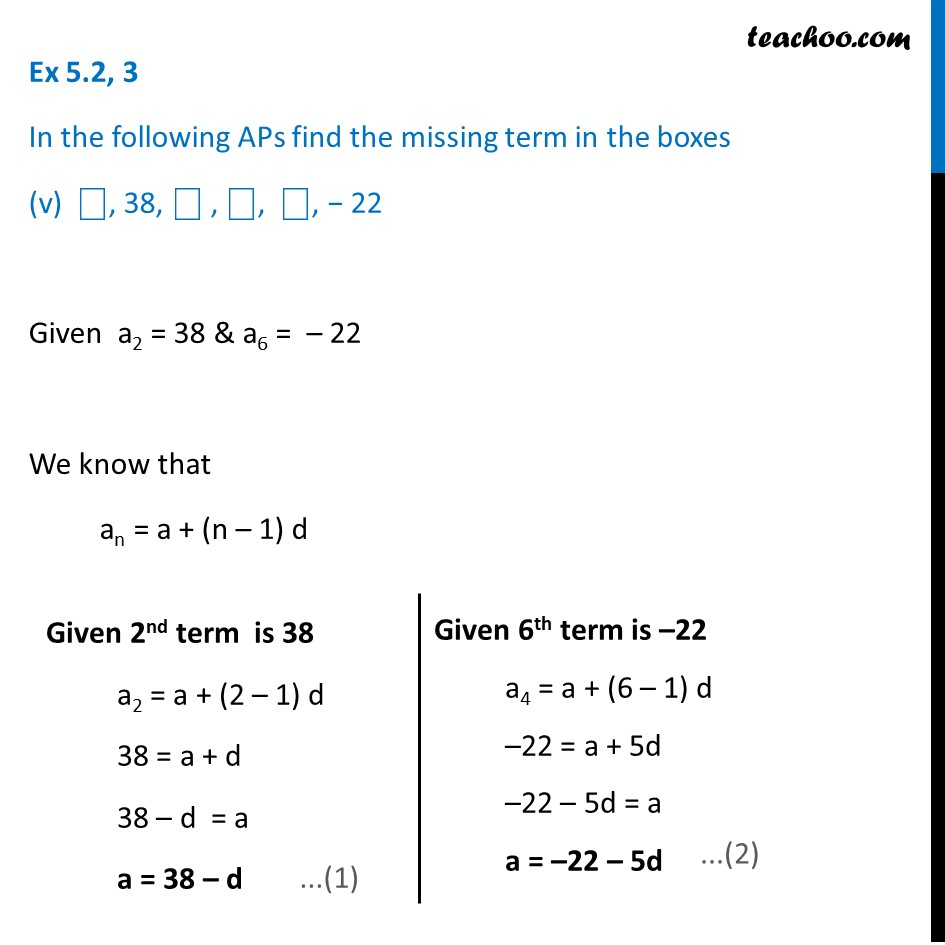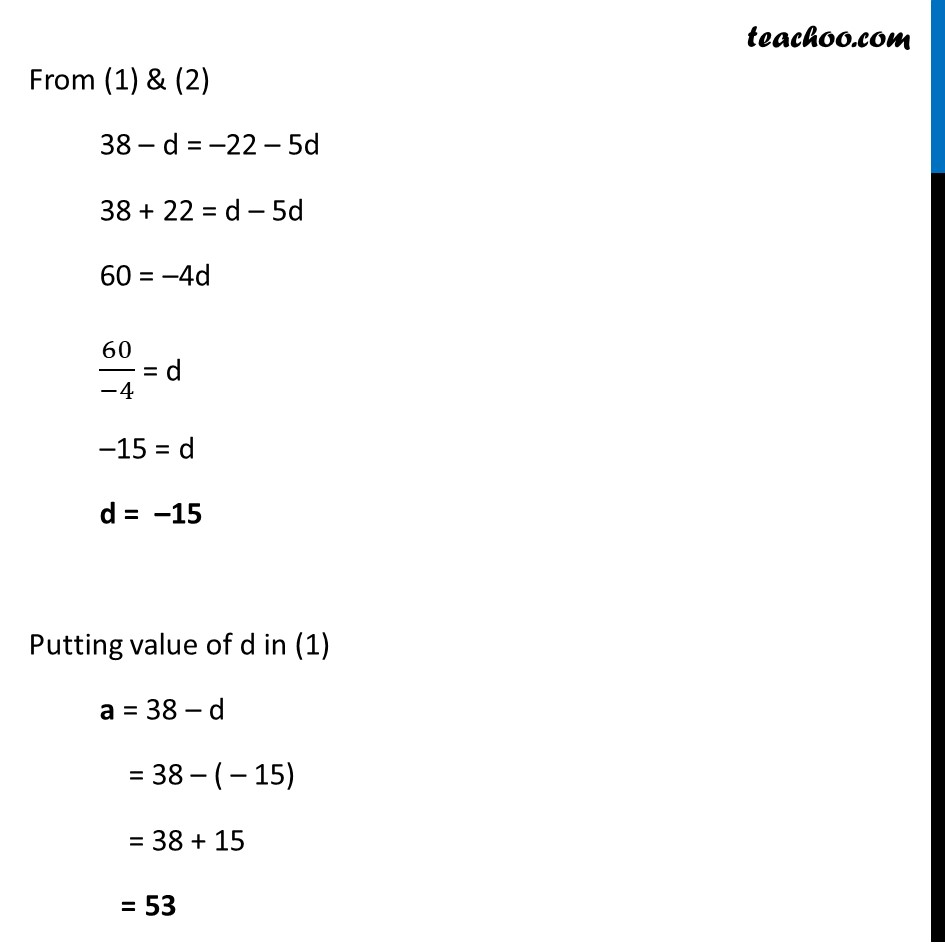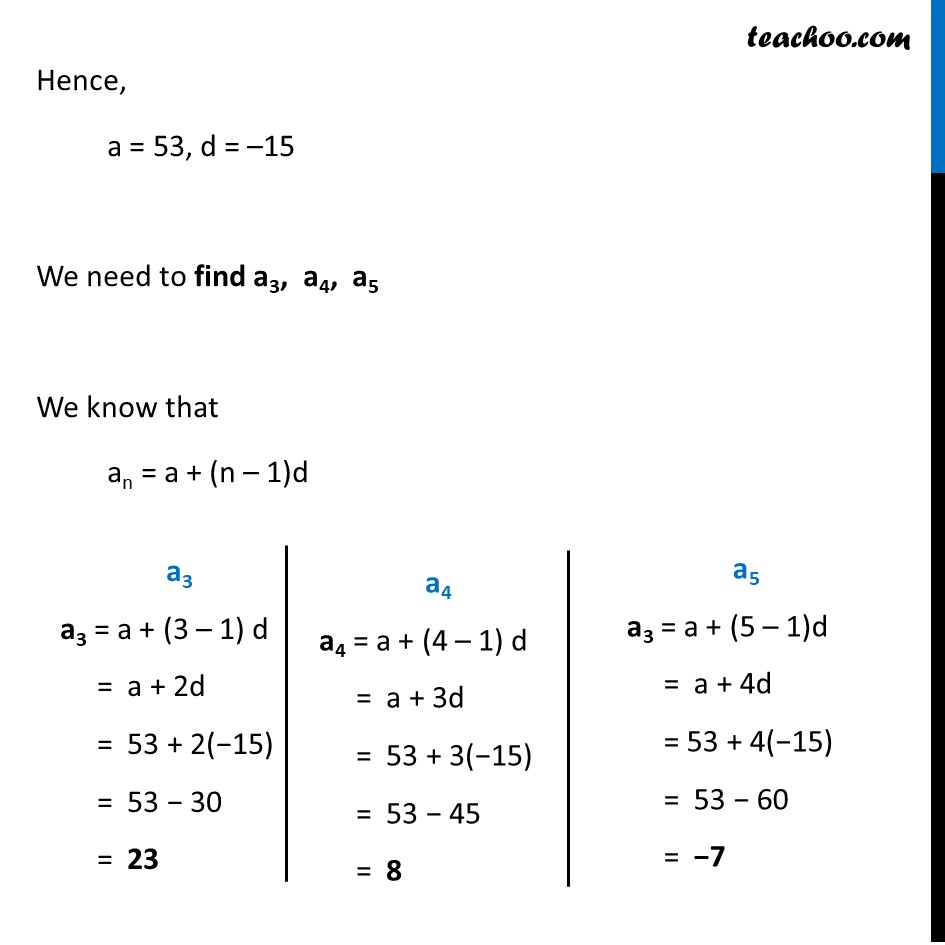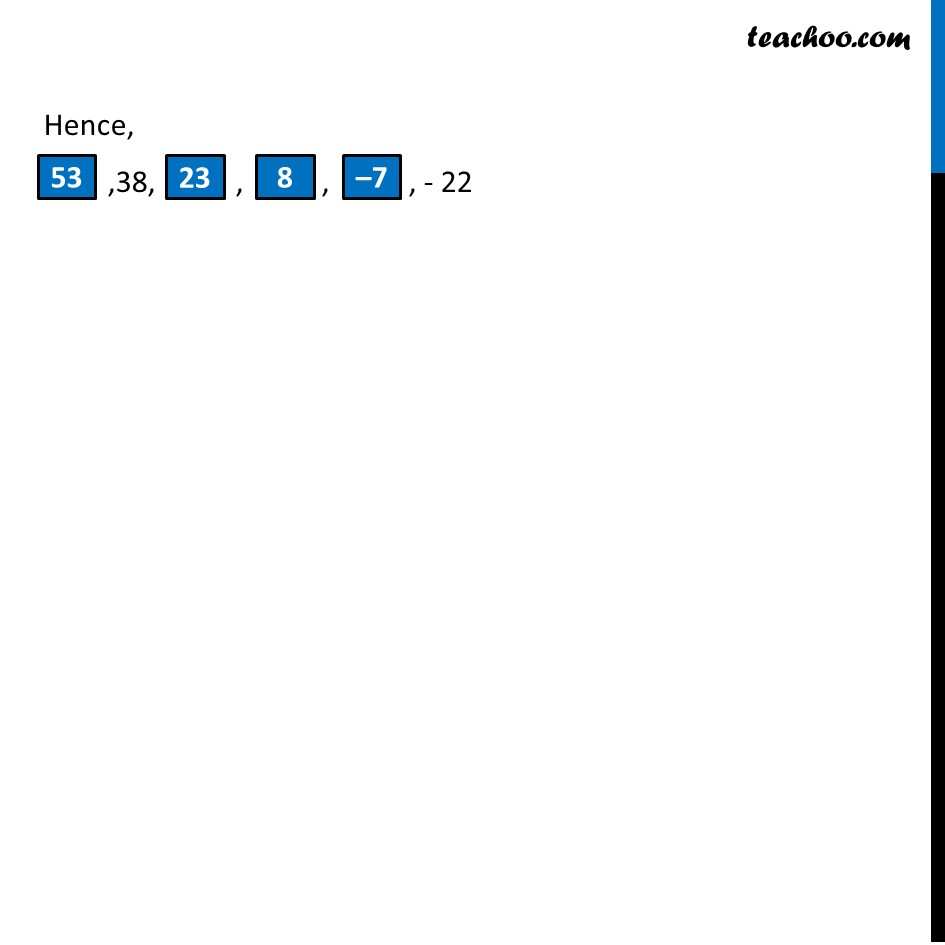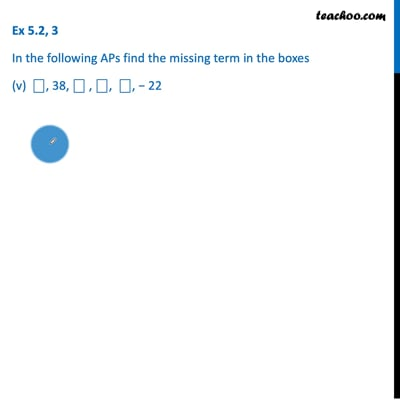This video is only available for Teachoo black users

Introducing your new favourite teacher - Teachoo Black, at only ₹83 per month

### Transcript

Ex 5.2, 3 In the following APs find the missing term in the boxes (v) ⎕, 38, ⎕ , ⎕, ⎕, − 22 Given a2 = 38 & a6 = – 22 We know that an = a + (n – 1) d Given 2nd term is 38 a2 = a + (2 – 1) d 38 = a + d 38 – d = a a = 38 – d Given 6th term is –22 a4 = a + (6 – 1) d –22 = a + 5d –22 – 5d = a a = –22 – 5d From (1) & (2) 38 – d = –22 – 5d 38 + 22 = d – 5d 60 = –4d 60/(−4) = d –15 = d d = –15 Putting value of d in (1) a = 38 – d = 38 – ( – 15) = 38 + 15 = 53 Hence, a = 53, d = –15 We need to find a3, a4, a5 We know that an = a + (n – 1)d a3 a3 = a + (3 – 1) d = a + 2d = 53 + 2(−15) = 53 − 30 = 23 a4 a4 = a + (4 – 1) d = a + 3d = 53 + 3(−15) = 53 − 45 = 8 a5 a3 = a + (5 – 1)d = a + 4d = 53 + 4(−15) = 53 − 60 = −7 Hence, ,38, , , , - 22